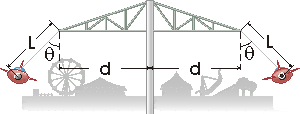# Amusement Park Ride - Angular Speed

#### FAJISTE

1. The problem statement, all variables and given/known data

In an amusement park rocket ride, cars are suspended from L = 4.37-m cables attached to rotating arms at a distance of d = 6.09 m from the axis of rotation. The cables swing out at an angle of θ = 53.9° when the ride is operating. What is the angular speed of rotation?2. Relevant equations

(mv^2)/r = mg
wr(angular speed) = v

3. The attempt at a solution

Okay, so first I calculated the total radius which was 6.09 m + (sin53.9)(4.37) and got a radius of 9.62 m. Then, since there is no normal force, the tension is equal to mg, thus making mv^2/r = mg. Masses cancel out and I got v^2/r = g I got a velocity of 9.7145 m/s. Using the angular speed equation : wr = v. For angular speed I got 1.01 deg / sec. Computer says this is wrong, what did I do wrong?

#### Delphi51

Homework Helper
the tension is equal to mg, thus making mv^2/r = mg
Errors here! You must separate the vertical forces from the horizontal ones.
The tension is partly vertical and partly horizontal, so you'll need sine and cosine.
Write two separate equations for the horizontal and vertical forces.
I expect you will have two unknowns to find with your two equations, though you are probably only interested in one of them.

#### FAJISTE

I only have one try left, and I'm almost positive that I use the velocity of the x component which utilizes the equation : v^2/r = tan53.9(g)

Is that correct?

I got angular speed of 1.18 deg/s which appears to still be wrong....sigh what am I doing wrong now?

#### Delphi51

Homework Helper
v^2/r = tan53.9(g) looks pretty good, though I have no idea how you could get it without separating the vertical and horizontal forces.

It is 1.18 radians/s for the angular velocity.

#### FAJISTE

Okay, I guess I'll have to ask my teach about it because 1.18 radians/s is wrong :(

#### FAJISTE

Thanks for the help though!

### The Physics Forums Way

We Value Quality
• Topics based on mainstream science
• Proper English grammar and spelling
We Value Civility
• Positive and compassionate attitudes
• Patience while debating
We Value Productivity
• Disciplined to remain on-topic
• Recognition of own weaknesses
• Solo and co-op problem solving